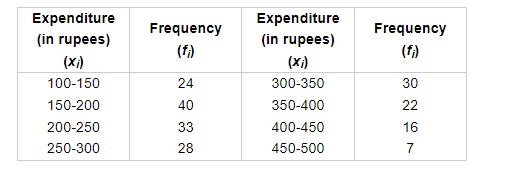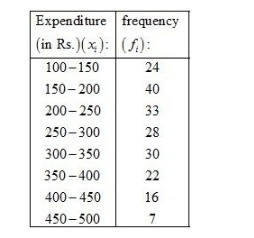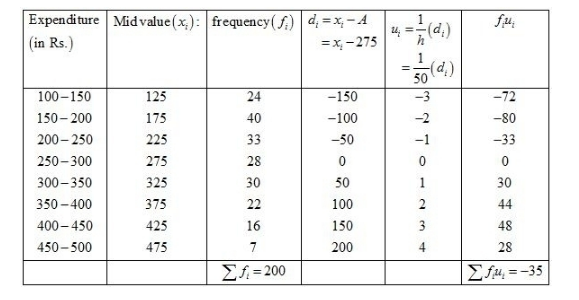# The following tables gives the distribution of total household

Question:

The following tables gives the distribution of total household expenditure (in rupees) of manual workers in a city.Find the average expenditure (in rupees) per household

Solution:

Given:Let the assumed mean be A = 275 and = 50.We know that mean, $\bar{X}=A+h\left(\frac{1}{N} \sum f_{i} u_{i}\right)$

Now, we have $N=\sum f_{i}=200, \sum f_{i} u_{i}=-35, h=50$ and $A=275$.

Putting the values in the above formula, we get

$\bar{X}=A+h\left(\frac{1}{N} \sum f_{i} u_{i}\right)$

$=275+50\left(\frac{1}{200} \times(-35)\right)$

$=275-\frac{1750}{200}$

$=275-8.75$

$=266.25$

Hence, the average expenditure (in Rs.) per household is $266.25$.